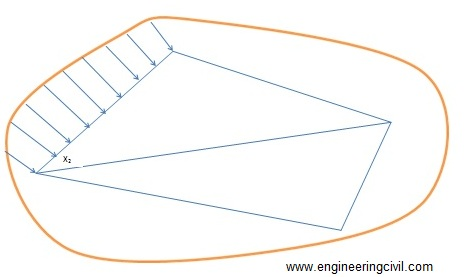Awarded as the best online publication by CIDC

# Computation of areas and volumes

Keywords: computation of area, computation volume, area calculation of irregular boundaries

This article discusses the methods which are used to determine the areas of lands and the volumes of earthwork (either cutting or filling). These methods find application in determining the areas of existing plots. Volume determination is useful to determine the volumes of cutting through elevated areas and volume of embankment for the purpose of laying routes through low lying areas.

Computation of areas
Area of land means the area as projected on a horizontal plane. The important point is,it is not the actual area of surface.
1) To determine the area of a plot with straight boundaries, the plot is then subdivided into areas having straight boundaries (i.e. triangles, rectangles or trapezium) and the area calculated using appropriated formulas for this subdivided plots.

2) To determine areas with irregular boundaries, the plot is then subdivided into areas (of regular polygons) leaving the strip of irregular land along the boundaries whose area is determined separately and added to the calculated of regular polygons.

Area is determined by two methods namely:
1. By direct use of field notes
2. From previously plotted plan

The first method for the computation of areas, i.e., the area determination using field notes is explained as follows:

Area determined by directly using the field notes
To determine the area using this method, it is not necessary to draw the whole plan to scale. Because the accuracy of the work is solely depend on the accuracy of the fieldwork, which is already done. In doing the fieldwork, as much of the whole area as possible is to be included for accuracy. The survey lines shouldlie down carefully for this.This is shown in figure 1 as follows:Figure 1: Planning of the survey lines for the determination of area

The calculation of the plot area consists of two parts namely:
i.Calculation of the areas of the skeletons
ii.Calculations of the area enclosed between the surveys lines of the skeleton and the irregular boundaries.

Determination of the areas of the skeletons
a. By geometry
The areas of the triangles forming skeletons may be calculated by the following formulae:
1) Area of triangle= ?[s(s-a)(s-b)(s-c)]
Where a, b and c denotes the sides of the triangle
Semi-perimeter, s = (a+b+c)/2

2) Area of triangle=bh/2
Where b is the length of any side and h is the perpendicular distance from this side.

b) Area by co-ordinates
This method is used in the case of traverse survey. In this method of survey, first, a set of points well distributed in the area to be measured is selected. These points are joined by survey lines from which perpendicular offsets may be taken to the boundary. The measurements to be taken in such a traverse survey are essentially the distance of the lines connecting the points and the angle between successive lines or the bearings of the lines (bearing is the angle between the north direction and the line, measures clockwise from north).

The step wise procedures are given below:

• Arrange the co-ordinates in an order.
• Find the sum of the product of the co-ordinates joined by full lines, and the sum of the products of the co-ordinates joined by broken lines.
• Find the difference between the sums
• This difference is equal to twice the area of the traverse.
• Half this difference =area of the traverse

Determination of the area enclosed between the surveys lines of the skeleton and the irregular boundaries
To determine the areas of strips of land along the foundation, the adjoining survey line is decided into intervals and the offset to the boundary is measured at each point. The areas of the irregular strips may now be calculated by multiplying the mean of each successive pair of adjacent offsets by the distance between them as shown in figure 2.

O1= offset at chainage X1

O2= Offset at chainage X2

Mean offset= (O1+O2)/2

Distance between the offsets = X2-X1

Area of the portion between the skeleton X2 and X1 and the boundary = {(O1+O2)/2}(X2-X1)Figure 2: Determination of the area along boundaries

The second method for the computation of area (i.e. computation of area from previously plotted plan) is discussed here.